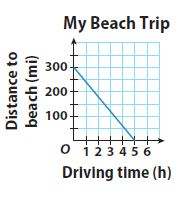Homework Explained - Math Practice 101Dear guest, you are not a registered member. As a guest, you only have read-only access to our books, tests and other practice materials.

As a registered member you can:

Registration is free and doesn't require any type of payment information. Click here to Register.
Go to page:
Chapter 5: Writing Linear Equations; Lesson 1: Writing Linear Equations from Situations and Graphs

Guided Practice

• Question 1

Li is making beaded necklaces. For each necklace, she uses 27 spacers, plus 5 beads per inch of necklace length. Write an equation to find how many beads Li needs for each necklace.

a. input variable:

• Question 1

b. output variable:

• Question 1

c. equation:

• Type below:
• Question 2

Kate is planning a trip to the beach. She estimates her average speed to graph her expected progress on the trip. Write an equation in slope-intercept form that represents the situation.• Type below:
• Question 3

At 59 °F, crickets chirp at a rate of 76 times per minute, and at 65 °F, they chirp 100 times per minute. Write an equation in slope-intercept form that represents the situation.

• Type below:

Essential Question Check-In

• Question 4

Explain what m and b in the equation $$\text{y} = \text{mx + b}$$ tell you about the graph of the line with that equation.

• Type below:

Yes, email page to my online tutor. (if you didn't add a tutor yet, you can add one here)